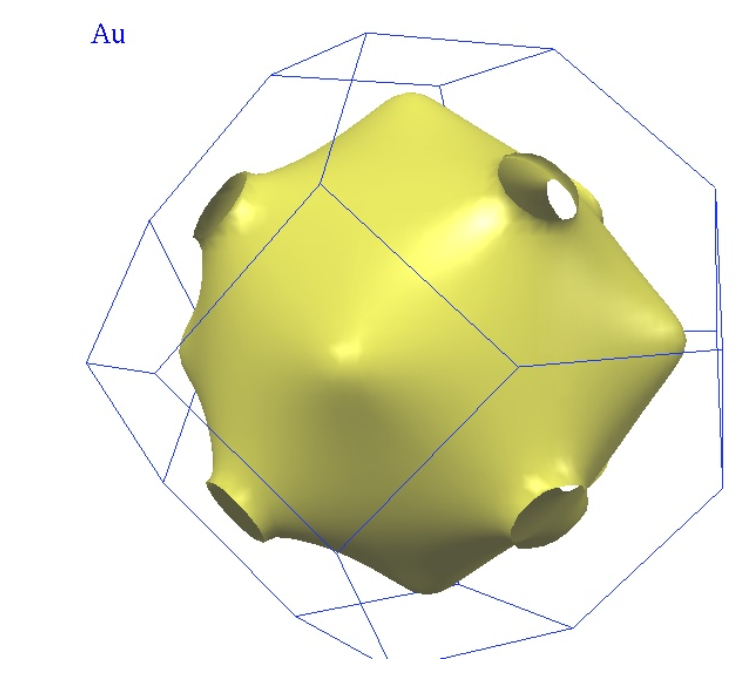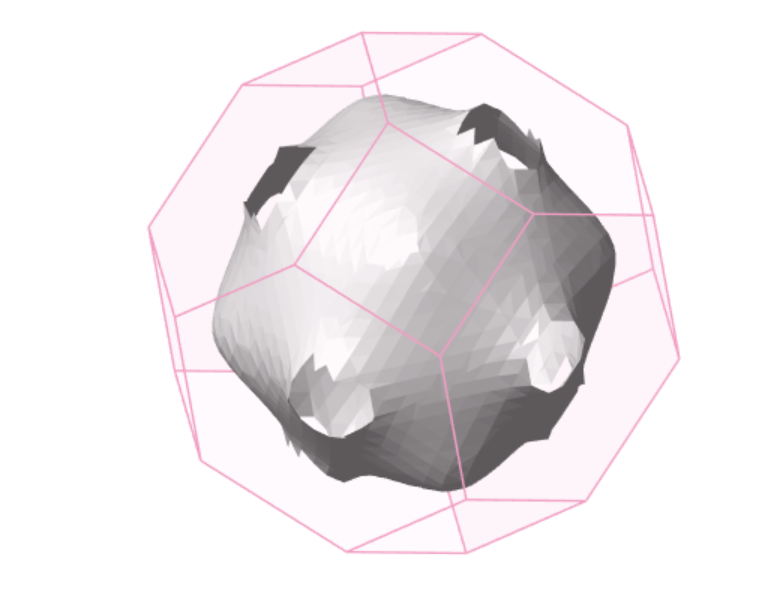# How to make the surface smoother?

My problem:

Recently, I am learning THREE.js to visualize a chemical structure, like this:However, in order to make the painted surface appeared more smooth, I choose to use as many triangles as possible which is stored in BufferGeometry data structure. This cause a big problem: the json file which stores the Triangular coordinates turn out to be very large even more than 200MB, which is bad for network transmission and it takes up large amount of storage space.

So, I wonder if there are any solutions in THREEJS to this problem? I once wonder whether I can use small amount of triangles’ coordinates, then use the interpolant to make more triangles, but there seems no example to illustrate the process of my assumption, so I find it hard to do this kind of experiment. This problem can be interpreted as how to make the surface in the below picture more smooth. In this way, I don’t need big file but can still visualize a smooth surface.This really confuse me for a long time, can anyone give me some suggestion? Thank you very much if you can~

I think you are looking for smooth / averaged normals (increasing the amount of triangles with flat shading enabled will not work, you’d have to subdivide the model until each triangle is approximately the size of a single pixel on any screen.)

(1) If you are using Blender to create models, you can simply select the model, press `F3` (or `space`) and search to `Shade Smooth`.

(2) If models are generated procedurally in three, you can try recalculating normals using Geometry.computeVertexNormals (it’s enough to do it only once, after you generate your geometry):

``````model.geometry.computeVertexNormals();
``````

Also, if you enable it somewhere on the way, make sure flatShading is off for the model material.

3 Likes

It seems like your model is exported with every triangle being individual rather than sharing vertices, so each face has it’s own perpendicular normals. It seems this issue is most common with the OBJ format, but not caused by it but the modelling software export.

You should try fix it from the software you created it or try use `yourGeometry = THREE.BufferGeometryUtils.mergeVertices( yourGeometry);` in order to connect trinagles that share the same position and `yourGeometry.computeVertexNormals()` afterwards.3 Likes

Thanks for your kind reply, but acutually my purpose is to make the surface be smoother, not to connect triangles that share the same position. Currently, I use the below code to store the geometry data : the variable “positions” store the triangles’ coordinates, the variable “normal” store the triangles’s normal vector.
`var positions = new Float32Array( numOftriangle * 3 * 3 ); var normals = new Float32Array( numOftriangle * 3 * 3 ); var geom = new THREE.BufferGeometry(); geom.addAttribute( 'position', new THREE.BufferAttribute( positions, 3 ) ); geom.addAttribute( 'normal', new THREE.BufferAttribute( normals, 3 ) );`
In this way, the more triangles’ coordinates, smoother the surface will be.
As you can see the current surface(below picture) not turns out to be smooth enough. I wonder how to make it more smoother, use interpolation or somewhat？

I didn’t use Blender to create models, just some amount of triangles’ coordinates.

Sharing vertices is highly recommended, it doesn’t change the geometry triangles count, every regular geometry does this unless for parts that are supposed to not share attributes like UV or normals.

Without sharing the vertices you won’t be able to use computeVertexNormals, you could only compute the normals yourself from the equation you created the geometry from, in case you did.

What you’re missing is an index, describing which attributes shape a triangle instead creating new for every triangle, you will also reduce memory a lot by this.

1 Like

Yes, if I didn’t misunderstand your meaning, do you mean that by sharing vertices, I can share some attributes for example, color for every triangles and reduce memory by this? However, this solution will not be able to smooth the surface unless I increase the number of triangles right? However now I only have a small amount of triangles’ coordinates, but I want the surface to be smoother. I don’t want to reduce memory when visualizing, but reduce the file size of the file which store the coordinates of triangles.

The current problem is that I cannot obtain more triangles’ vertex coordinates to achieve the result that each triangle is approximately the size of a single pixel on any screen.

There is absolutely no need for more triangles, there are more than enough already. Smoothing here means nothing else than the normals being weighted/continuous, the same for every vertex at a position (hence why you shouldn’t waste memory here), which in your geometry is not the case, they are perpendicular for each individual triangle.

So asides of saving memory you’ll get a smooth surface as well, you can try this and see the result:

``````yourGeometry = THREE.BufferGeometryUtils.mergeVertices( yourGeometry);
yourGeometry.computeVertexNormals();
``````

You don’t need mergeVertices if you build the index yourself when building the geometry, computing normals yourself isn’t always possible and what happens in computeVertexNormals is a very common general approach to compute the average normals for smooth shading.

To understand normals better you can look at the normals helper example, the red lines indicate the direction the normals are aligned to, you see on curved surfaces like a sphere there is only 1 at a position pointing at some average direction, while for a cube there are 3 at a position as you want a sharp edge for each face of the cube.

Normals visualized: https://threejs.org/examples/?q=normal#webgl_geometry_normals

4 Likes

Just to clarify - “subdividing triangles until each is a size of a pixel” was absolutely not serious - that’s why both @Fyrestar and I mention that increasing triangle amount is not the right way to go.

3 Likes

Thanks~
Then I modified my code:

``````    var geom = new THREE.BufferGeometry();

geom.addAttribute( 'position', new THREE.BufferAttribute( positions, 3 ) );
// geom.addAttribute( 'normal', new THREE.BufferAttribute( normals, 3 ) );
geom.addAttribute( 'color', new THREE.BufferAttribute( colors, 3 ) );

geom.computeBoundingSphere();

geom = THREE.BufferGeometryUtils.mergeVertices(geom);
geom.computeVertexNormals();
``````

But there appears an error:

But the geom has been defined already, do you know what happens?

BufferGeometryUtils isn’t part of the core, you need to include it from the examples folder:
`examples/js/utils/BufferGeometryUtils.js`

worked perfectly! thank you so muchBy the way, do you know how to make it much smoother? The edge of the hollow appears not mild, there are the sharp corners of triangles.

It’s hard to tell from just looking at it, can you share the geometry? Judging by the first image it might be too close vertices causing sharp details and not matching triangle vertices.

You might increase the tolerance parameter in mergeVertices.

I can only provide the vertices’ coordinates of triangles:
vertices_cart_fs.json (2.8 MB)
And below code shows how I created the geometry use the information provided by the json file above.

``````for(var i = 0; i < vertices_cart_fs.length; i++){
for(var j = 0; j < vertices_cart_fs[i].length; j++){
var plane = vertices_cart_fs[i][j];

var ax = plane*fsScale;
var ay = plane*fsScale;
var az = plane*fsScale;
var bx = plane*fsScale;
var by = plane*fsScale;
var bz = plane*fsScale;
var cx = plane*fsScale;
var cy = plane*fsScale;
var cz = plane*fsScale;

positions[ k ]     = ax;
positions[ k + 1 ] = ay;
positions[ k + 2 ] = az;

positions[ k + 3 ] = bx;
positions[ k + 4 ] = by;
positions[ k + 5 ] = bz;

positions[ k + 6 ] = cx;
positions[ k + 7 ] = cy;
positions[ k + 8 ] = cz;

// colors
if(plane == 0){
color.setRGB(237/255, 237/255, 101/255);//yellow
}else if(plane == 1){
color.setRGB(204/255, 25/255, 204/255);//purple
}else if(plane == 2){
color.setRGB(0/255, 204/255, 204/255);//light blue
}else if(plane == 3){
color.setRGB(204/255, 25/255, 0/255);//red
}else if(plane == 4){
color.setRGB(25/255, 204/255, 69/255);//green
}else if(plane == 5){
color.setRGB(25/255, 25/255, 204/255);//dark blue
}
colors[ k ]     = color.r;
colors[ k + 1 ] = color.g;
colors[ k + 2 ] = color.b;

colors[ k + 3 ] = color.r;
colors[ k + 4 ] = color.g;
colors[ k + 5 ] = color.b;

colors[ k + 6 ] = color.r;
colors[ k + 7 ] = color.g;
colors[ k + 8 ] = color.b;

k = k + 9;
}
}

var geom = new THREE.BufferGeometry();

geom.setAttribute( 'position', new THREE.BufferAttribute( positions, 3 ) );
geom.setAttribute( 'color', new THREE.BufferAttribute( colors, 3 ) );

geom.computeBoundingSphere();

geom = THREE.BufferGeometryUtils.mergeVertices(geom);
geom.computeVertexNormals();
``````

It seems that I change the tolerance parameter in mergeVertices to 0.001, 0.01 and 1, as the default number is 0.0001, however nothing changed after I increased the mergeVertices.